﻿ 船体结构强度评估的线性设计波法研究
 舰船科学技术2019, Vol. 41Issue (4): 34-38PDF

Research on linear design wave method of structure strength evaluating of hull
XIA Feng, HUANG Dong, TENG Hong-yuan, GUO Jian-jun
Wuhan Second Ship Design and Research Institute, Wuhan 430064, China
Abstract: According to the special ship type cannot be suited for the formula of rules to calculate the wave load of the hull, takes a FPSO for an example, introducing a long term prediction of the design wave method, using the design wave method to determine the extreme long-term prediction for wave load and design wave parameters of the FPSO. Furthermore, a comparative analysis is given to the formula of rules and nonlinear correction of wave loads. Finally, an evaluate is given to the structure strength of the FPSO by using the design wave load result. The analysis results show that the calculation of the wave loads of FPSO by the long-term prediction of the design wave method has a certain of rationality,and the structure strength of the FPSO is satisfied for the rules.
Key words: wave loads     design wave method     FPSO     long-term prediction     structure strength
0 引　言

1 设计波参数确定方法 1.1 载荷控制参数

1.2 波浪载荷传递函数（RAO）计算

1.3 长期预报

 $P({{X}} > {{x}}) = \frac{{\mathop \Sigma \limits_i \mathop \Sigma \limits_j \mathop \Sigma \limits_k \mathop \Sigma \limits_l {N_ * }{p_i}{p_j}{p_k}{p_l}{P_ * }({{X}} > {{x}})}}{{\mathop \Sigma \limits_i \mathop \Sigma \limits_j \mathop \Sigma \limits_k \mathop \Sigma \limits_l {N_ * }{p_i}{p_j}{p_k}{p_l}}}\text{。}$

 ${P_ * }({{X}} > x) = {\rm{exp}}\left\{ - \frac{{{x^2}}}{{2{{({{{m}}_0})}_*}}}\right\} \text{。}$

 $N = \left\{ \mathop \Sigma \limits_i \mathop \Sigma \limits_j \mathop \Sigma \limits_k \mathop \Sigma \limits_l {N_ * }{p_i}{p_j}{p_k}{p_l}\right\} \times T \times 3\ 600/\left\{ \mathop \Sigma \limits_i \mathop \Sigma \limits_j \mathop \Sigma \limits_k \mathop \Sigma \limits_l {p_i}{p_j}{p_k}{p_l}\right\}\text{，}$

 $\frac{1}{{P(X > \mathop x\limits^ \wedge (N)}} = N\text{。}$
1.4 设计波参数确定

2 计算结果与分析 2.1 目标船体与计算条件表 1 目标船的主要参数 Tab.1 Major parameter of the target ship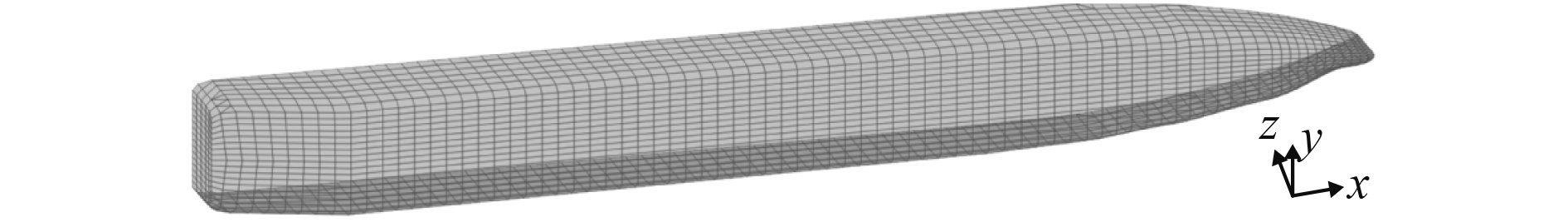图 1 目标船水动力模型 Fig. 1 Hydrodynamic model of the target ship
2.2 计算结果 2.2.1 载荷响应RAO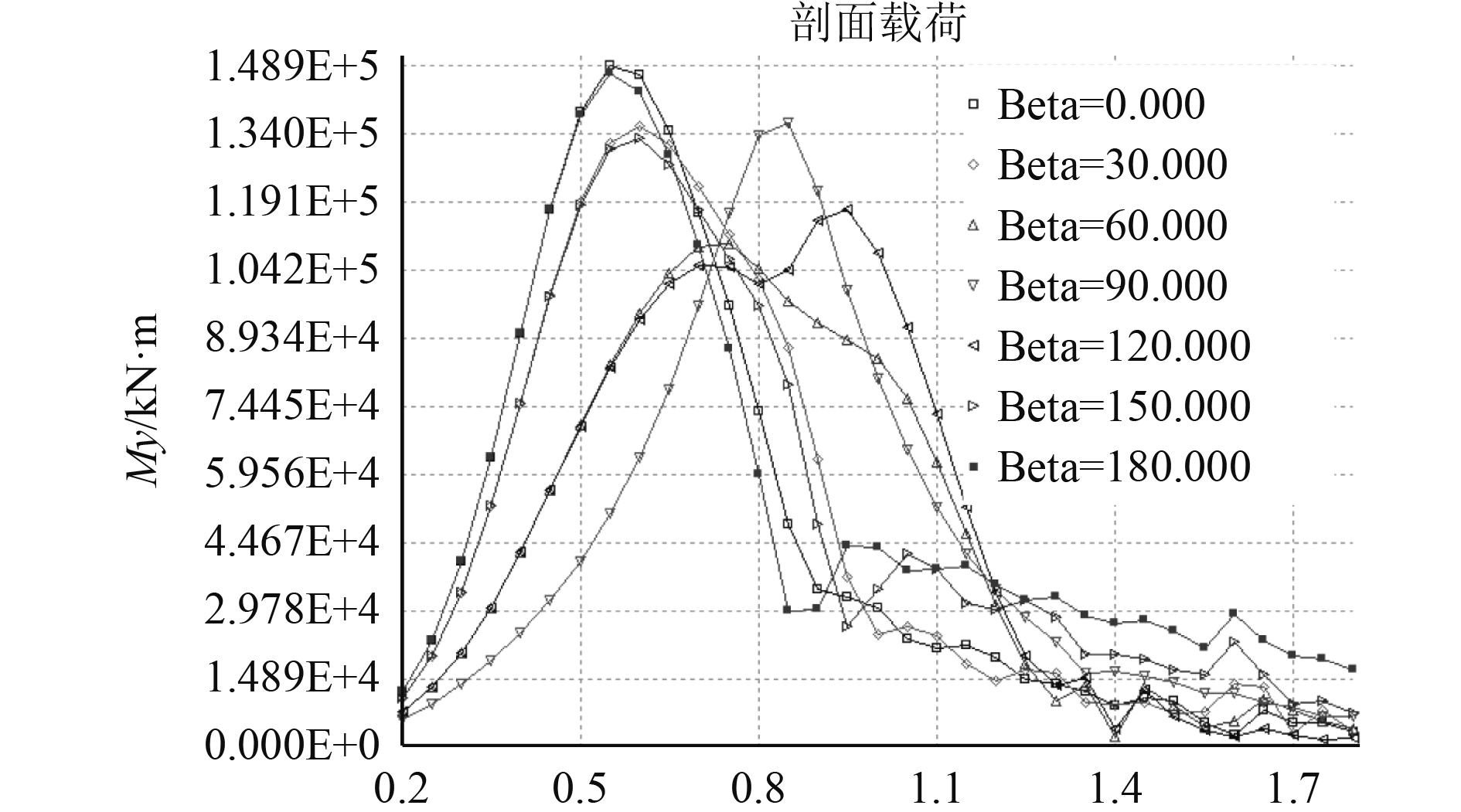图 2 船中垂向波浪弯矩RAO Fig. 2 Vertical wave moment RAO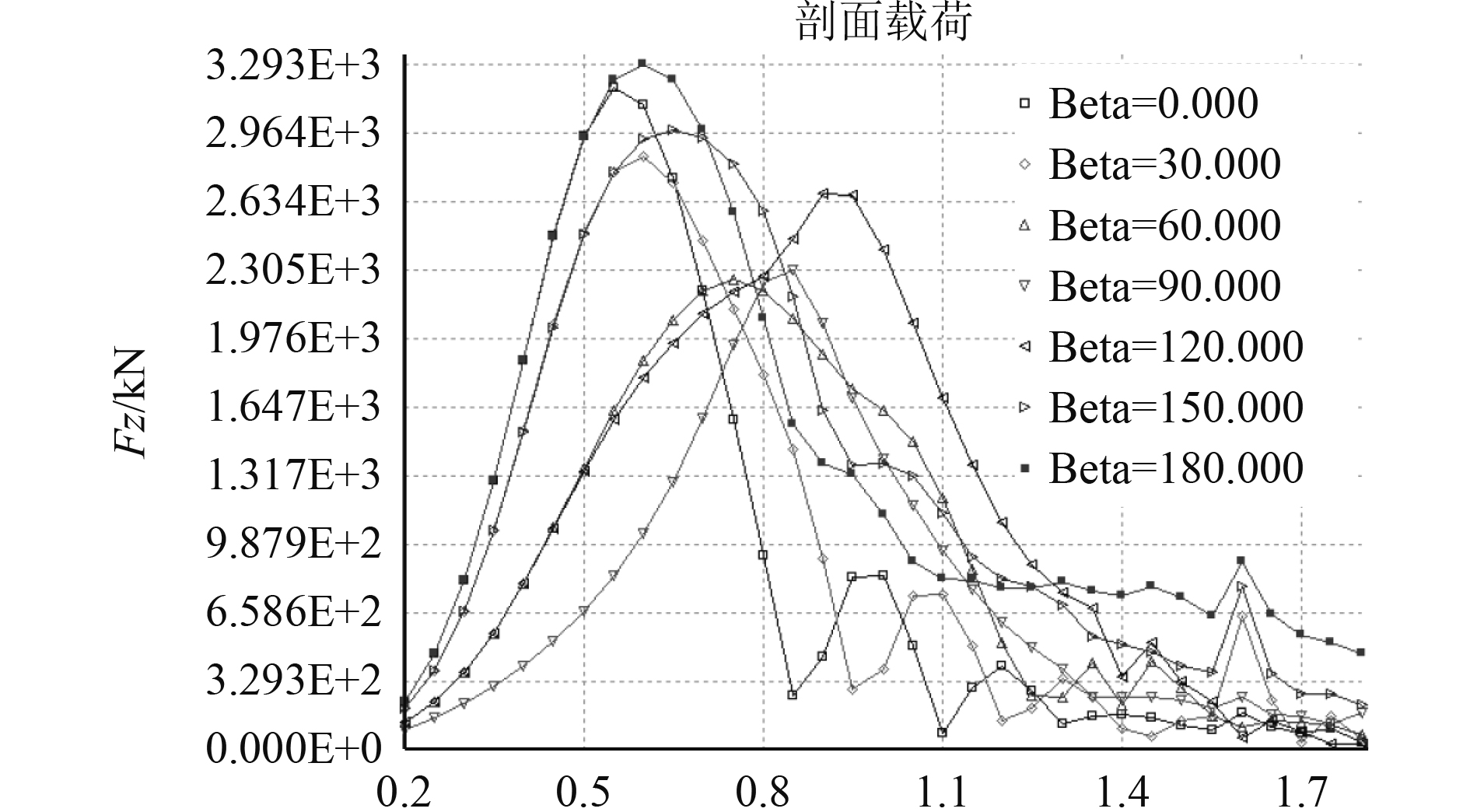图 3 最大剖面垂向剪力RAO Fig. 3 Maximum profile vertical shear force RAO
2.2.2 长期预报结果

1）波浪谱选取为Jonswap谱；

2）波浪形式考虑短峰波的影响；

3）扩散函数为 $\displaystyle\frac{2}{{\text{π}}}{\cos ^2}\theta$

4）海浪散布图为渤海海域波浪散布图；

5）超越概率为重现期百年一遇海况下的超越概率。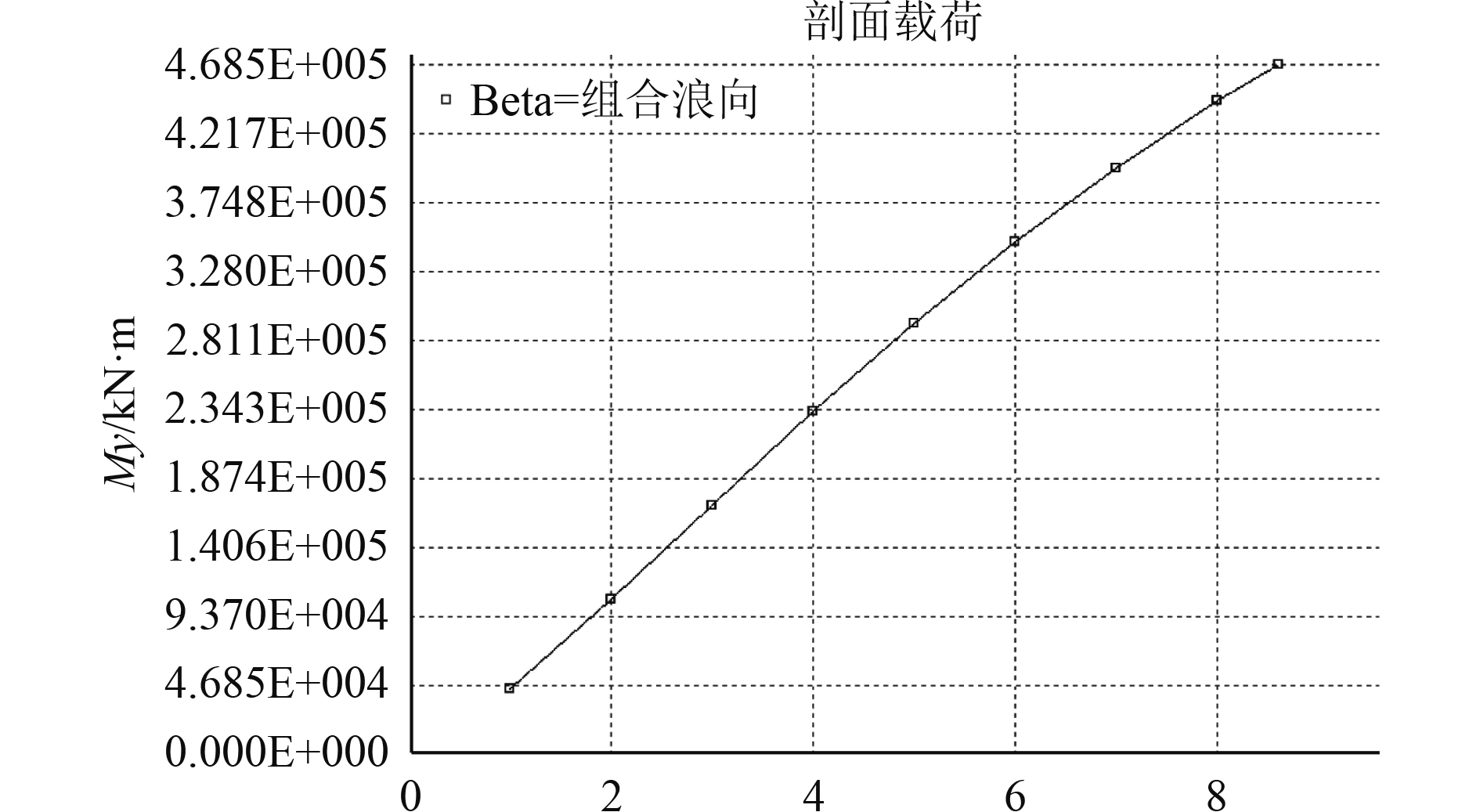图 4 船中垂向波浪弯矩长期预报极值 Fig. 4 Long-term forecast extreme value of vertical wave bending moment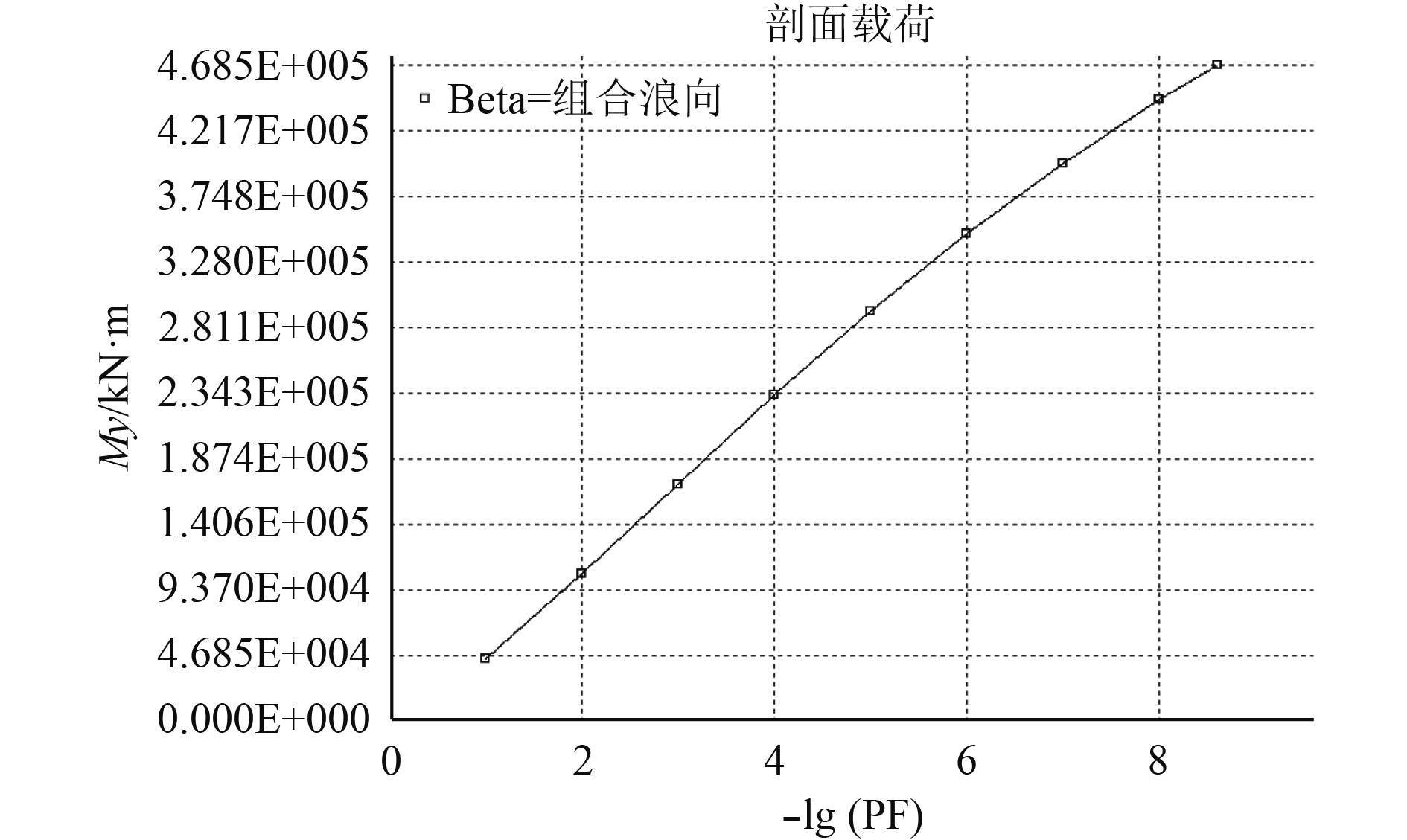图 5 最大剖面垂向剪力长期预报极值 Fig. 5 Long-term forecast extreme value of maximum profile vertical shear force
2.2.3 设计波结果表 2 设计波波浪参数 Tab.2 Parameters of the design wave

2.2.4 规范计算结果表 3 目标船波浪载荷计算对比 Tab.3 A comparison about the wave load calculation of target ship
2.3 波浪载荷计算结果分析

 ${f_{nl - h}} = \frac{{190{C_b}}}{{95{C_b} + 55({C_b} + 0.7)}}\text{，}$
 ${f_{nl - s}} = \frac{{110({C_b} + 0.7)}}{{95{C_b} + 55({C_b} + 0.7)}}\text{。}$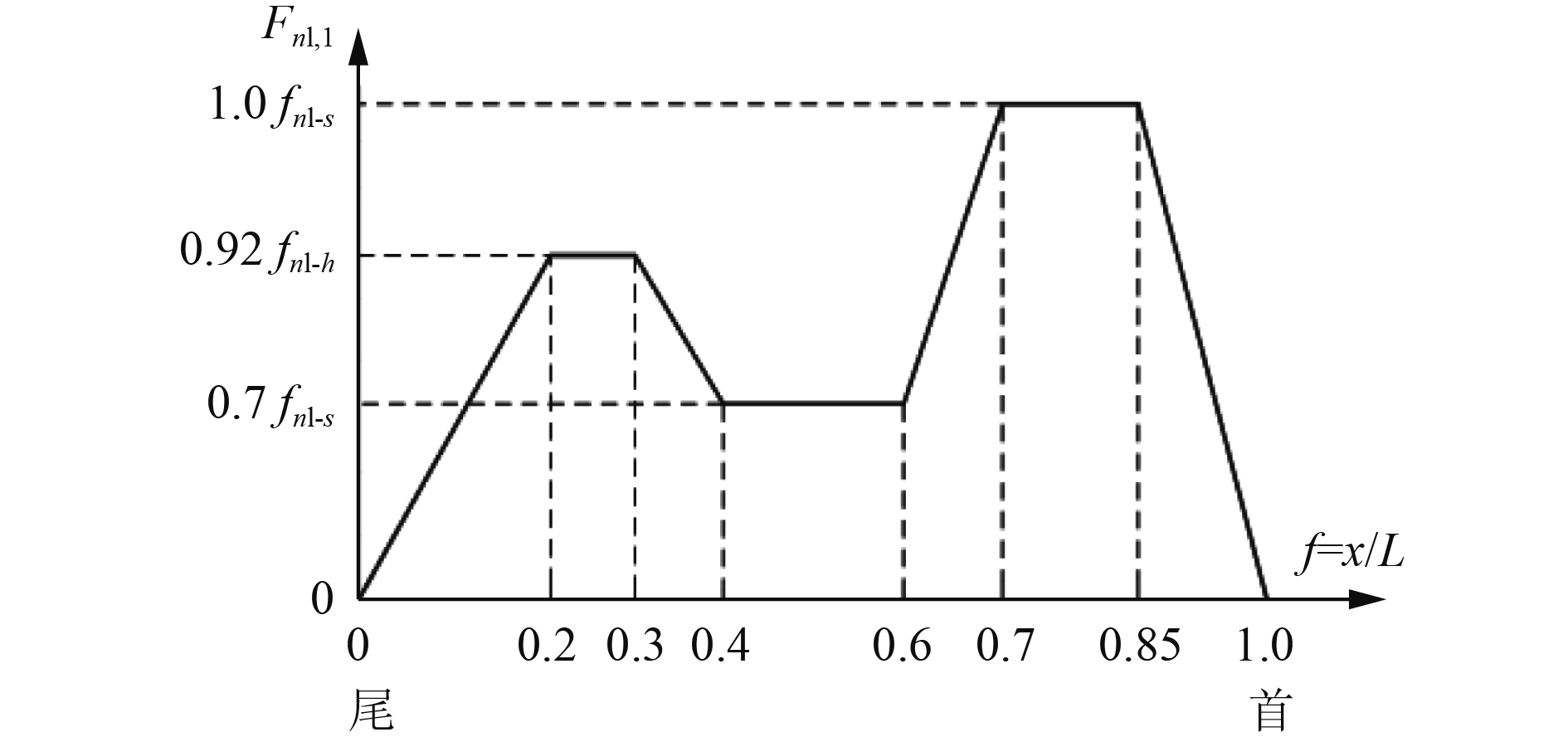图 6 系数 ${f_{nh - 1}}$ 沿船长分布 Fig. 6 The coefficient ${f_{nh - 1}}$ distributed along the length of the ship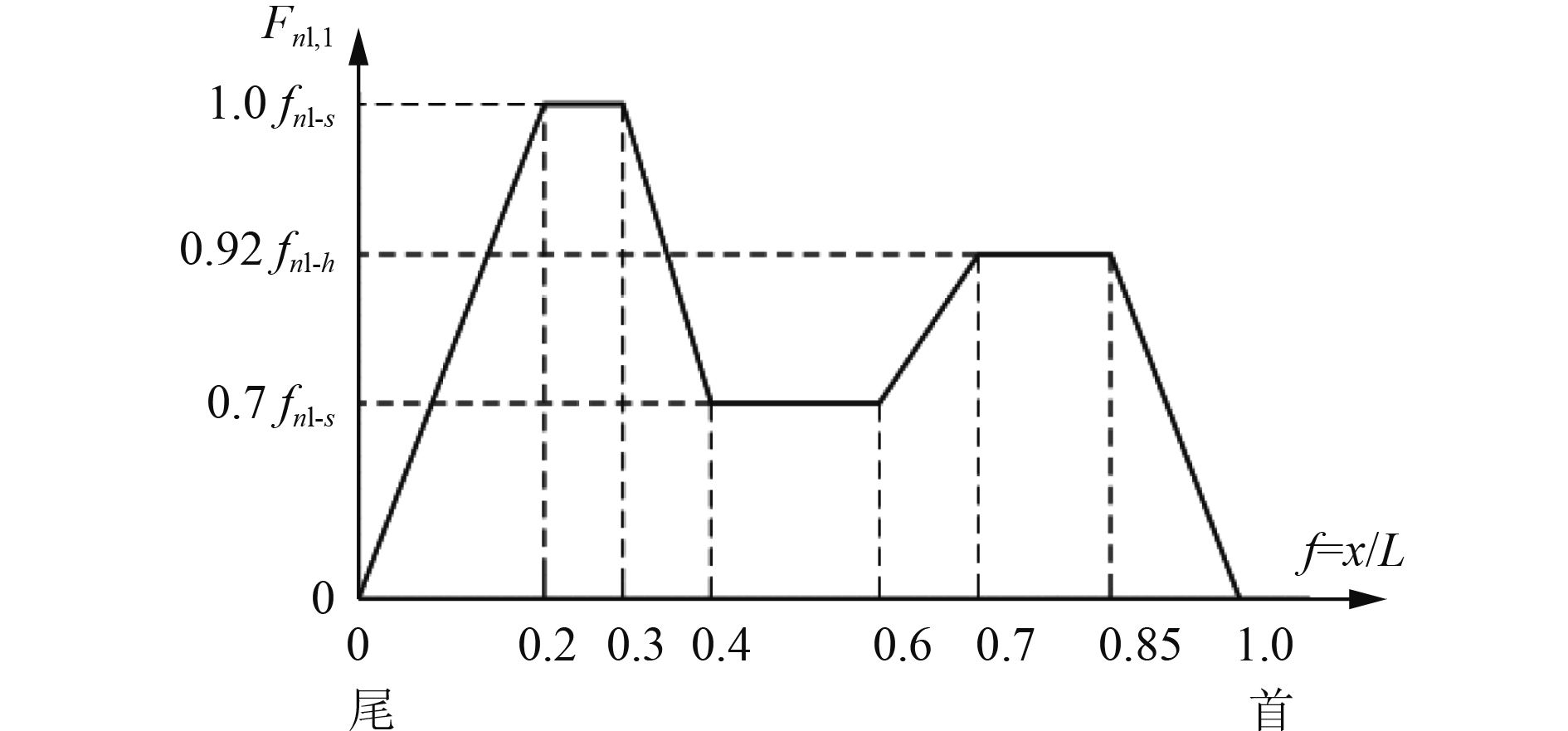图 7 系数 ${f_{nh - 1}}$ 沿船长分布 Fig. 7 The coefficient ${f_{nh - 1}}$ distributed along the length of the ship

3 船体结构强度评估 3.1 模型建立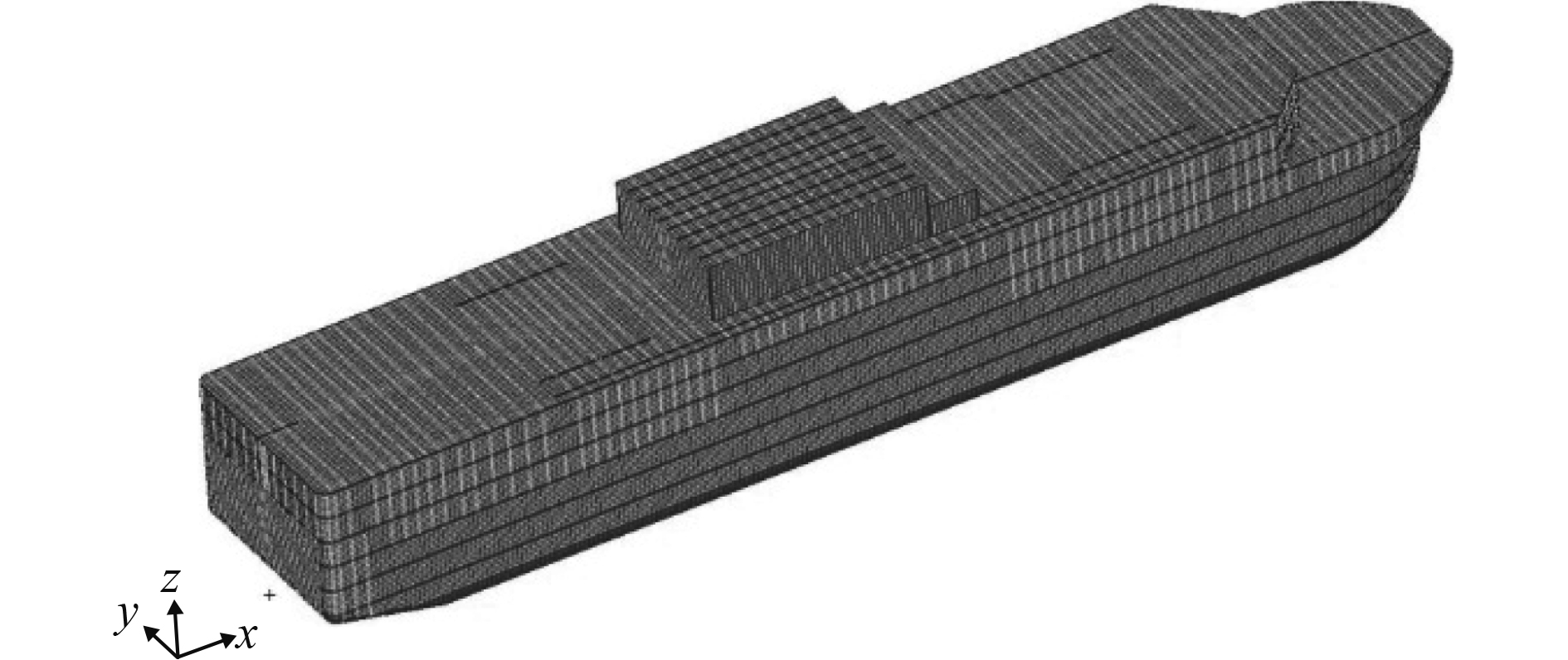图 8 全船有限元模型 Fig. 8 The finite element model of whole ship
3.2 计算载荷施加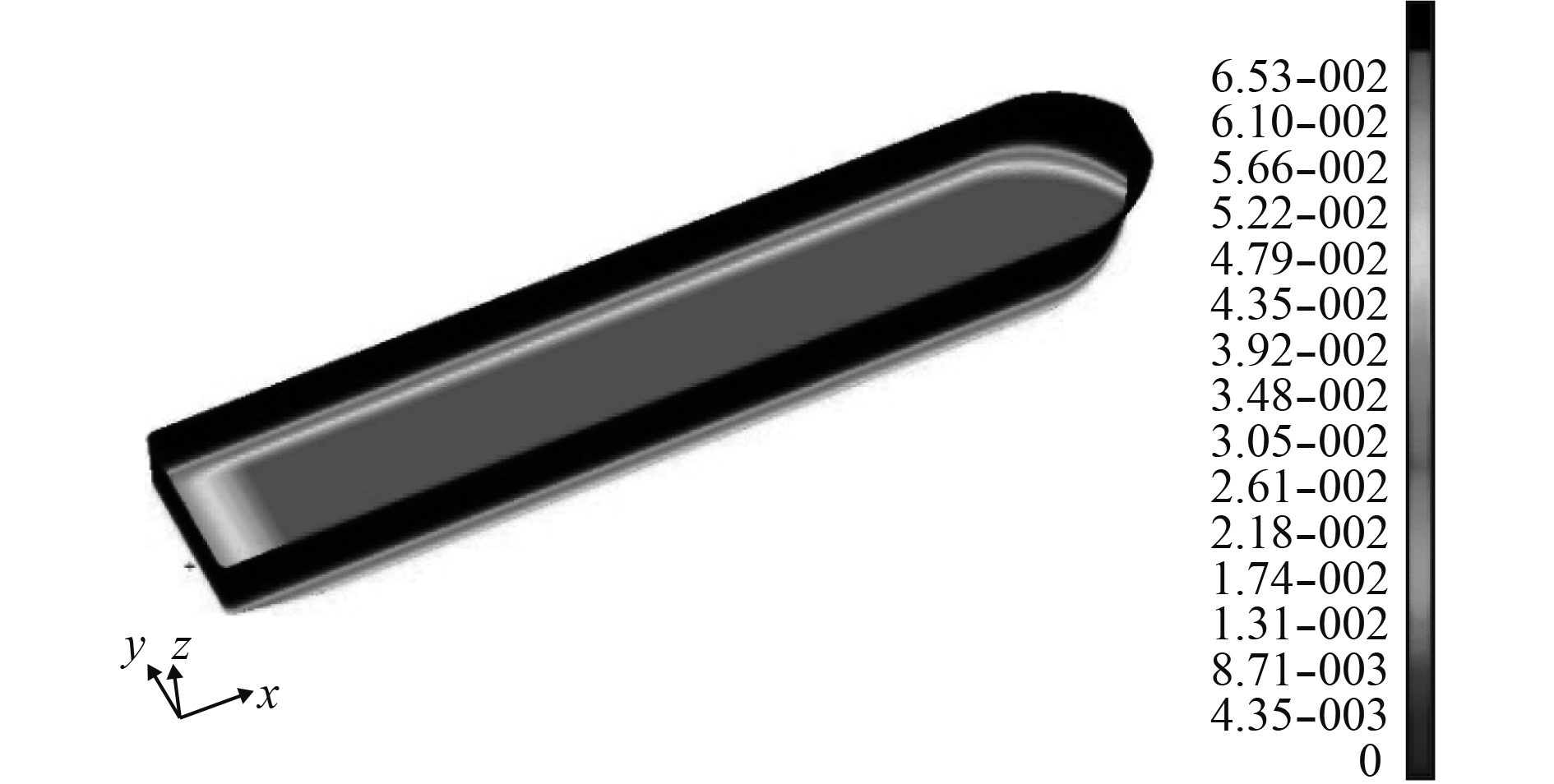图 9 舷外静水压力云图 Fig. 9 The hydrostatic pressure cloud picture of outshell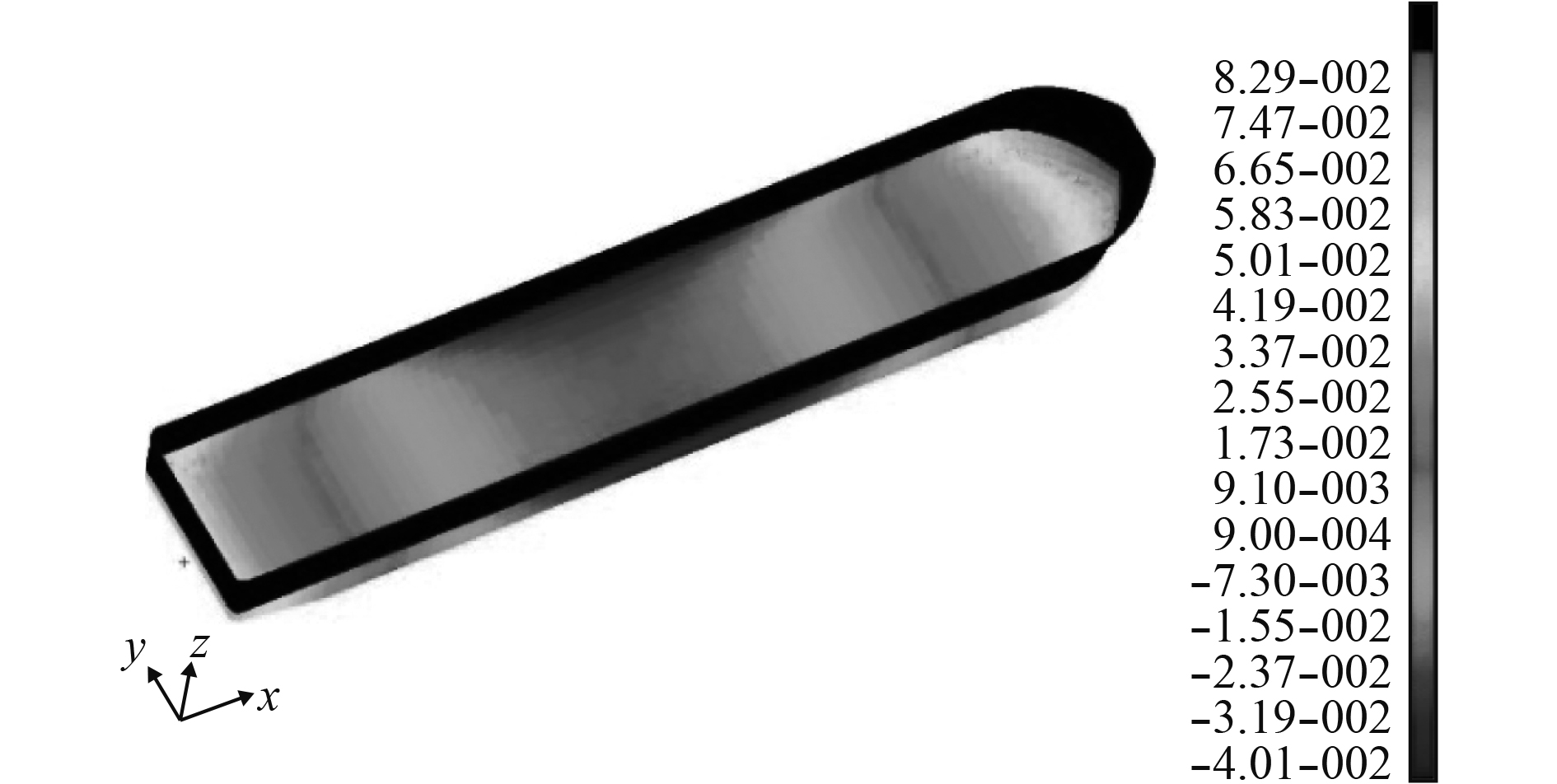图 10 舷外波动压力云图 Fig. 10 The dynamic pressure cloud picture of outshell
3.3 边界条件

3.4 评估结果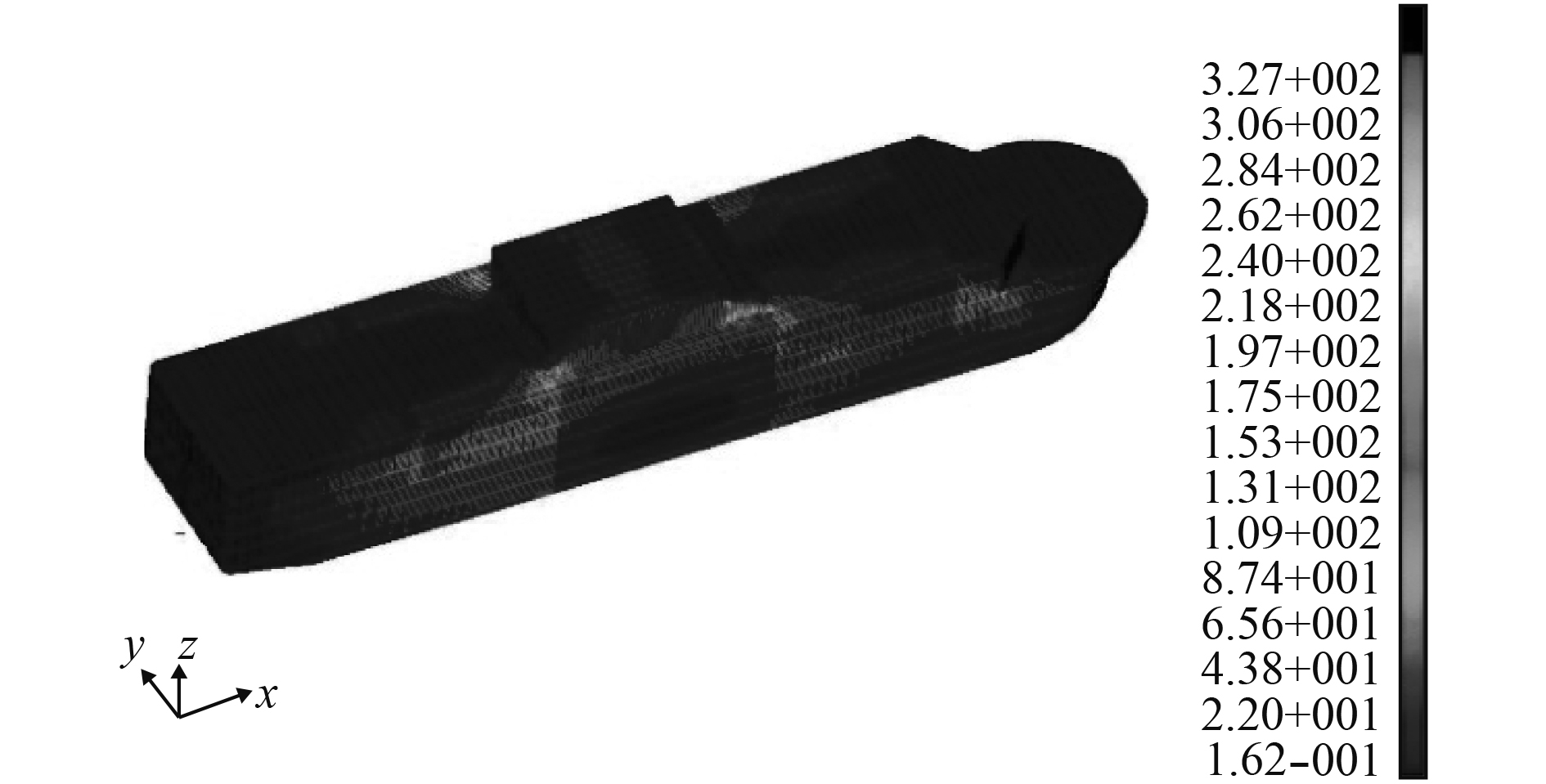图 11 极限工况下整船应力云图 Fig. 11 The stress cloud picture of whole ship under extreme condition表 4 主要构件应力结果 Tab.4 The stress results of main component
4 结　语

1）用线性设计波法计算的波浪载荷比规范计算法得到的波浪载荷稍大，这是因为规范中波浪载荷的计算公式适用于L<500 m，L/B>5，B/D<2.5， ${C_b} \geqslant 0.6$ 的常规船型，而目标船L/B=3.78超出了规范计算公式的使用要求，需要采用直接计算法计算其波浪载荷，采用线性设计波法计算的目标船波浪载荷更为保守。

2）CCS《钢质海船入级规范（2015）》中给定了对于线性设计波法直接计算波浪载荷的非线性修正公式，波浪载荷非线性修正结果与线性设计波直接计算结果相差不大，用线性设计波法能较好的预报目标船这类方形系数较大、非线性效应不显著的船型。

3）由船体结构强度评估结果可以看出，在不考虑应力集中的情况下，极限工况下的船体主要构件的应力水平满足规范对总纵强度的要求。

  中国船级社. 海上浮式装置入级规范[S]. 北京: 人民交通出版社, 2014.  中国船级社. 钢质海船入级规范[S]. 北京: 人民交通出版社, 2015.  方钟圣. 西北太平洋波浪统计集[M]. 北京:国防工业出版社, 1996.  戴仰山, 沈进威. 船舶波浪载荷[M]. 北京: 国防工业出版社, 2007.  张朝阳, 刘俊, 白艳彬. 深水半潜平台波浪载荷计算的设计波方法研究[J]. 中国海洋平台, 2012, 27(5): 34-40. DOI:10.3969/j.issn.1001-4500.2012.05.008